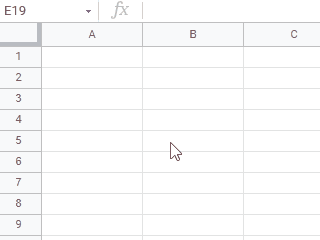GistsHere, I would like to introduce a report for occurring and resolving the infinite loop on Google Spreadsheet using Google Apps Script. I have reported this to Google issue tracker. Ref

## Sample script: Occurring infinite loop

This sample script is a test script for confirming the infinite loop. Please be careful this. Please copy and paste the following script to the script editor of Google Spreadsheet and save it. And, please install OnChange trigger to the function onChange().

And, please put a custom function of =sample(). By this, the infinite loop is started as showing in the above demonstration.

function sample() {
return new Date();
}

// This function is installed as the OnChange trigger. And please put a custom function of =sample() to a cell.
// By this, the infinite loop is started.
function onChange(e) {
const formula = "=sample";
const tempFormula = "=sampleFormula";
[
[formula, tempFormula],
[tempFormula, formula],
].forEach(([a, b]) =>
e.source
.createTextFinder("^\\" + a)
.matchFormulaText(true)
.useRegularExpression(true)
.replaceAllWith(b)
);
}


### Supplement

This sample script can be used for refreshing the functions on the cells, for example, when the sheet is added and sheet name is renamed.

For example, when OnEdit trigger is used, the infinite loop doesn’t occur. So when you want to refresh the functions in the cells by editing the cells, you can use this script with OnEdit trigger (both simple and installable triggers).

## Principle of this infinite loop

In the above sample script, when the OnChange trigger is fired, the function of onChange() is run. This is the correct result.

But, when the script of onChange() replaced the custom function, the OnChange trigger is fired again. By this cycle, this infinite loop occurs.

When I had tested this, it seems that the infinite loop is sometimes stopped by a bug that the formula is not replaced from =sampleFormula to =sample. But this is not the essence of the issue of this infinite loop.

### My proposal

Here, at 1st firing onChange(), it occurs by the manual operation. But at 2nd firing onChange(), it occurs by the script.

By this, I thought that when changeType has a value of SCRIPT, this infinite loop can be avoided.

### Workaround

In the current stage, when a workaround I proposed to the following script is used, this issue can be avoided.

This sample script is a workaround for avoiding the infinite loop. Please copy and paste the following script to the script editor of Google Spreadsheet and save it. And, please install OnChange trigger to the function onChange().

And, please put a custom function of =sample(). In this case, the custom function is refreshed only one time. The infinite loop doesn’t occur.

function sample() {
return new Date();
}

// This function is installed as the OnChange trigger. And please put a custom function of =sample() to a cell.
function onChange(e) {
const prop = PropertiesService.getScriptProperties();
if (!prop.getProperty("run")) {
const formula = "=sample";
const tempFormula = "=sampleFormula";
[
[formula, tempFormula],
[tempFormula, formula],
].forEach(([a, b]) =>
e.source
.createTextFinder("^\\" + a)
.matchFormulaText(true)
.useRegularExpression(true)
.replaceAllWith(b)
);
prop.setProperty("run", "done");
} else {
prop.deleteProperty("run");
}
}


## Note

• For the top of script, when OnEdit trigger is used, the infinite loop doesn’t occur.